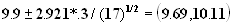LEGACY CONTENT. If you are looking for Voteview.com, PLEASE CLICK HERE

This site is an archived version of Voteview.com archived from University of Georgia on May 23, 2017. This point-in-time capture includes all files publicly linked on Voteview.com at that time. We provide access to this content as a service to ensure that past users of Voteview.com have access to historical files. This content will remain online until at least January 1st, 2018. UCLA provides no warranty or guarantee of access to these files.

Probability and Statistics                      Name__________________________
Spring 1997 Flex-Mode 45-733
Practice Final
Keith Poole

(10 Points)

1. Suppose we take a random sample of size n from a normal distribution with mean m and variance 81. Determine the minimum sample size, n, such that the absolute difference between the sample mean and m is less than .2 with probability at least .95.

P[ |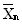- m | < 0.2] ³ 0.95
P[-.02 <- m < 0.2} = P[ -(0.2/9)n1/2< (- m)n1/2/9<(0.2/9)n1/2] =
F [+(0.2/9)n1/2 ] - F [-(0.2/9)n1/2 ] = 2F [(0.2/9)n1/2 ] - 1 ³ 0.95
Þ F [(0.2/9)n1/2 ] ³ 0.975
So (0.2/9)n1/2 ³ 1.96
n ³
7780

Probability and Statistics                      Name__________________________
Spring 1997 Flex-Mode 45-733
Practice Final Exam
Keith Poole

(10 Points)

2. A random sample of 101 is drawn from a normal distribution and it is found that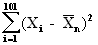= 887

Construct 95% and 99% confidence limits for s2.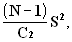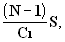or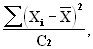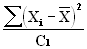for 95% limits a = 0.05, a/2 = 0.025, df = 100

C1 = 74.2219, C2 = 129.561

Þ (887/129.561) and (887/74.2219) = (6.846, 11.951)

For 99% limits a = 0.1, a/2 = 0.005, df = 100

C1 = 67.3276, C2 = 140.169

Þ (887/140.169) and (887/67.3276) = (6.328, 13.174)

Probability and Statistics                      Name__________________________
Spring 1997 Flex-Mode 45-733
Practice Final Exam
Keith Poole

(10 Points)

3. Pitt and GSIA students independently take an identical Prob-Stat I midterm examination. Assume the scores in both populations are normally distributed. You draw a sample of 8 GSIA students and obtain the scores: 72, 95, 83, 80, 95, 83, 80, 90; and you draw a sample of 9 Pitt students and obtain the scores: 74, 94, 88, 78, 60, 71, 66, 90, 83. Test the null hypothesis that the variances of the two populations are the same against the alternative hypothesis that the variances are not the same (a = .01).

GSIA       Pitt
N
= 8     M = 9= 84.75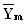= 78.22
S12 = 64.5 S22 = 132.19

H0:s 2g = s 2pitt
H1: s 2g¹ s 2pitt

Reject if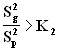or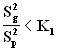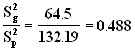K2 = 7.69
K1 = 1/(8.68)=0.1152

Since 0.1152 < 0.488 < 7.69

DO NOT REJECT H0

Probability and Statistics                      Name__________________________
Spring 1997 Flex-Mode 45-733
Practice Final Exam
Keith Poole

(10 Points)

4. Suppose you draw a random sample of size 13 from the CMU faculty and find that the average age is 49 years with sample standard deviation of 15 years. Assume that age is normally distributed. Construct the best possible decision rule for the hypothesis test:

H0: m = 56
H1: m ¹ 56

Do you accept or reject the null hypothesis (a = .05).

Reject ifor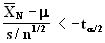a/2 = 0.025, 12df, t.025 = 2.179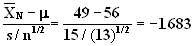Since -2.179<-1.683<2.179

DO NOT REJECT H0

Probability and Statistics                      Name__________________________
Spring 1997 Flex-Mode 45-733
Practice Final Exam
Keith Poole

(5 Points)

5. Suppose that the proportion of defective resistors in a large shipment to our plant is known to be .008. We take a random sample of 1000 resistors. What is the probability that less than 5 are defective.

l =np= (1000)(.008)=8

P(X<5)=F(4)=0.1

Probability and Statistics                      Name__________________________
Spring 1997 Flex-Mode 45-733
Practice Final Exam
Keith Poole

(10 Points)

6. Suppose we have the continuous probability distribution

f(x) =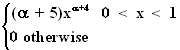Find the maximum likelihood estimator for a .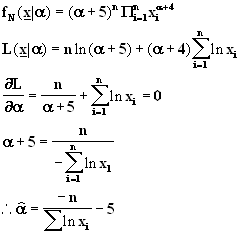Probability and Statistics                      Name__________________________
Spring 1997 Flex-Mode 45-733
Practice Final Exam
Keith Poole

(10 Points)

7. Suppose you draw a random sample of 8 first year masters students and find that their ages are 24, 29, 31, 29, 28, 28, 26, 32, respectively; and you draw a random sample of 6 second year masters students and find that their ages are 33, 31, 26, 27, 28, 30, respectively. Assume age is normally distributed in both populations with equal variance. Construct 95% confidence limits for the difference in mean ages of first and second year masters students.

1st : N=8,=28.375, S12=6.554

2nd: M=6,=29.167, S22=6.697

a =0.5, t.025=2.179, 12df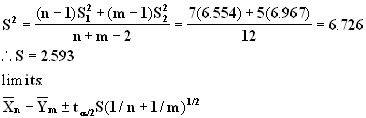So (28.375-29.267)± (2.179)(2.593)(1/8+1/6)1/2

(-3.843, 2.259)

Probability and Statistics                      Name__________________________
Spring 1997 Flex-Mode 45-733
Practice Final Exam
Keith Poole

(10 Points)

8. Suppose we have the bivariate continuous probability function

f(x,y) =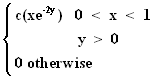a. Find c.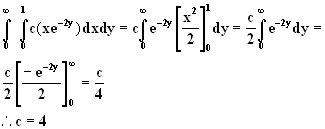b. Are X, Y independent.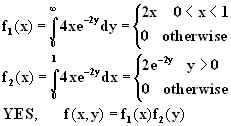Probability and Statistics                      Name__________________________
Spring 1997 Flex-Mode 45-733
Practice Final Exam
Keith Poole

(10 Points)

9. Suppose we know that exactly one of the following hypotheses must be true:

H0: s 2 = 350
H1: s 2 = 550

The population is normally distributed and the sample size is 30. How large must the sample variance be for you to reject the null hypothesis with a = .05.

Reject if (n-1)s2/s 02 > C2
a = 0.05, C2 = 42.5569, 29df

Hence (29s2)/350 > 42.5569 or s2 > 513.618

Probability and Statistics                      Name__________________________
Spring 1997 Flex-Mode 45-733
Practice Final Exam
Keith Poole

(10 Points)

10. A manufacturer of a machine to fill paint cans claims that her machine will fill paint cans at a given weight with a standard deviation of .7 ounce. You take a random sample of 14 filled paint cans and find that s = .88. Perform the following hypothesis test:

H0: s 2 = .49
H1: s 2 ¹ .49

Assume that the measurements are normally distribution and use a = .05.

Reject if (n-1)s2/s 02 > C2 or (n-1)s2/s 02 < C1

a = 0.05, C1 = 5.00874, C2 = 24.7356, 13df

Hence (n-1)s2/s 02 = 13(0.88)(0.88)/(0.49)=20.545

Since 5.00874 < 20.545 < 24.7356

DO NOT REJECT

Probability and Statistics                      Name__________________________
Spring 1997 Flex-Mode 45-733
Practice Final Exam
Keith Poole

(10 Points)

11. Suppose that the number of cars arriving at a drive through bank machine is governed by a Poisson process with a mean of 3 cars every 5 minutes. What is the probability that no more than 4 cars will arrive in a 5 minute period.

l =3, X = Number of Cars ,

P[X£ 4] = F(4) = 0.815

Probability and Statistics                      Name__________________________
Spring 1997 Flex-Mode 45-733
Practice Final Exam
Keith Poole

(10 Points)

12. The waiting times for customers at a local store are independent random variables with a mean of 3.0 minutes and a standard deviation of 2.0 minutes. Use the Central Limit Theorem to find the approximate probability that 100 customers can be served in less than 4 hours 30 minutes at this store.

m = 3, s = 2, N = 100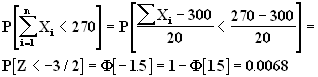Probability and Statistics                      Name__________________________
Spring 1997 Flex-Mode 45-733
Practice Final Exam
Keith Poole

(10 Points)

13. Suppose we have an urn containing 1000 balls numbered from 000 to 999. Six balls are drawn one at a time from the urn with replacement; that is, a ball is drawn, its numbered noted, and it is placed back in the urn. What is the probability that exactly two of the six numbers are the same and the other four are different.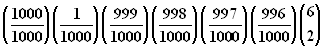Probability and Statistics                      Name__________________________
Spring 1997 Flex-Mode 45-733
Practice Final Exam
Keith Poole

(10 Points)

14. A large shipment of memory chips is delivered to our computer manufacturing plant. We take a random sample of 100 chips and find that 8 are defective. Compute 95% confidence limits for p, the true proportion of defectives.

Central Limit Theorem, 1 - a = 0.95, a/2 = 0.025, Z0.025 = 1.96

p ± 1.96 (.08*0.92/100)1/2
0.08 ± 0.053 = (.027, .133)

Probability and Statistics                      Name__________________________
Spring 1997 Flex-Mode 45-733
Practice Final Exam
Keith Poole

(10 Points)

15. Seventeen capacitors were randomly selected from the output of a process supposedly producing 10-picofarad capacitors. The 17 capacitors actually showed a sample mean of 9.9 picofarads and a sample standard deviation of .3 picofarads. Find 95% and 99% confidence limits for the true capacitance of the capacitors produced by this process. Assume that the measurements are normally distributed.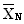= 9.9, n = 17, df = 16, s = .3

Confidence limits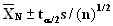a = .05, t0.025 = 2.12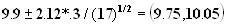a = .01, t0.005 = 2.921